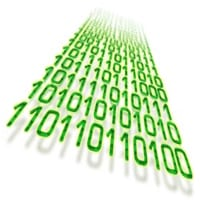# PLC Fiddle Math Challenge

PLC programming will often involve math equations that need to be solved. Every manufacturer of programmable logic controllers will include math logic for you to use in your programs. Numbering systems play an important role. You can usually perform math using BCD (Binary Coded Decimal), Binary or Floating Point. Here is a link that will review the numbering systems in the PLC:
What everyone ought to know about PLC numbering systemsPLC Fiddles provides four basic BCD math and a move function for our circuits. Add, Subtract, Multiply Divide and Move. We will look at these math instructions and show you the parameters required to program them. Our math challenges will help you gain a good understanding of how we can use math in the PLC. Trying these challenges with PLC Fiddle is fun and will help you with PLC programming.
Let’s get started. Continue Reading!